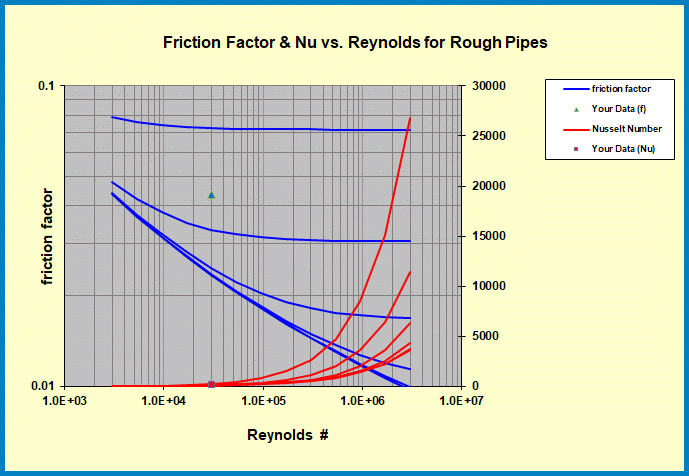# Forced Convection in Rough Pipes

UPDATED: 09/18/2022

This macro-enabled Excel workbook shows the calculation of a heat transfer coefficient for forced convection in rough pipes using the analogy between momentum and heat transfer.    The Colebrook  equation is used for the frictional pressure drop in non-smooth pipes and the Gnielinski correlation is used for the convection coefficient.   Inputs are the Reynolds number and relative roughness (ε/D) of the pipe.   The Colebrook equation is implicit in friction factor and thus Excel’s solver routine is implemented.  With the friction factor thus available, the Gnielinski correlation is used to find the corresponding Nusselt number (=hD/k).Frictional pressure drop coefficient and Nusselt number as a function of Reynolds number and relative roughness for forced convection in rough pipes

The HTT_pipe executable is limited to smooth pipes but allows thermally developing flows.   The current spreadsheet assumes fully developed (both thermally and hydrodynamically) conditions.